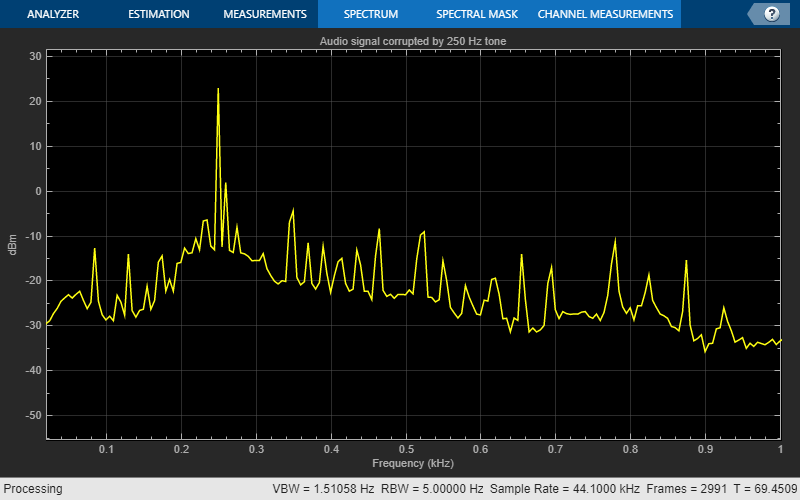# Desktop Real-Time Audio Acceleration with MATLAB Coder

This example shows how to accelerate a real-time audio application using C code generation with MATLAB® Coder™. You must have the MATLAB Coder™ software installed to run this example.

### Introduction

Replacing parts of your MATLAB code with an automatically generated MATLAB executable (MEX-function) can speed up simulation. Using MATLAB Coder, you can generate readable and portable C code and compile it into a MEX-function that replaces the equivalent section of your MATLAB algorithm.

This example showcases code generation using an audio notch filtering application.

### Notch Filtering

A notch filter is used to eliminate a specific frequency from a signal. Typical filter design parameters for notch filters are the notch center frequency and the 3 dB bandwidth. The center frequency is the frequency at which the filter has a linear gain of zero. The 3 dB bandwidth measures the frequency width of the notch of the filter computed at the half-power or 3 dB attenuation point.

The helper function used in this example is `helperAudioToneRemoval`. The function reads an audio signal corrupted by a 250 Hz sinusoidal tone from a file. `helperAudioToneRemoval` uses a notch filter to remove the interfering tone and writes the filtered signal to a file.

You can visualize the corrupted audio signal using a spectrum analyzer.

```reader = dsp.AudioFileReader('guitar_plus_tone.ogg'); scope = dsp.SpectrumAnalyzer('SampleRate',reader.SampleRate, ... 'RBWSource','Property','RBW',5, ... 'PlotAsTwoSidedSpectrum',false, ... 'SpectralAverages',10, ... 'FrequencySpan','Start and stop frequencies', ... 'StartFrequency',20, ... 'StopFrequency',1000, ... 'Title','Audio signal corrupted by 250 Hz tone'); while ~isDone(reader) audio = reader(); scope(audio(:,1)); end```### C Code Generation Speedup

Measure the time it takes to read the audio file, filter out the interfering tone, and write the filtered output using MATLAB code.

```tic helperAudioToneRemoval t1 = toc; fprintf('MATLAB Simulation Time: %d\n',t1)```
```MATLAB Simulation Time: 3.701829e+00 ```

Next, generate a MEX-function from `helperAudioToneRemoval` using the MATLAB Coder function, `codegen` (MATLAB Coder).

`codegen helperAudioToneRemoval`

Measure the time it takes to execute the MEX-function and calculate the speedup gain with a compiled function.

```tic helperAudioToneRemoval_mex t2 = toc; fprintf('Code Generation Simulation Time: %d\n',t2)```
```Code Generation Simulation Time: 2.167587e+00 ```
`fprintf('Speedup factor: %6.2f\n',t1/t2)`
```Speedup factor: 1.71 ```

## SupportGet trial now